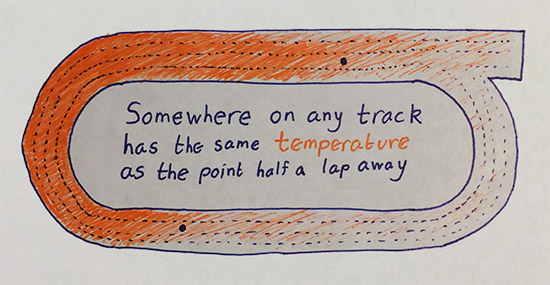Cartoons of cool ideas

from science and mathematicsTrack Temperatures

On any track, at any time, there must be a point that has exactly the same temperature as a point exactly half a lap away. This may be very surprising, and it is true for any temperature distribution on the track, provided that the temperature is a continuous function. That is, the temperature can not instantaneously jump values at any point. In the cartoon above, temperature is represented by a shade of orange. More orange corresponds to a higher temperature. The two blue dots are half a lap apart from each other, and they are at equal temperature values.

To prove this fact, you can use the Intermediate Value Theorem. Consider the function that represents the difference in temperature between a point and the point half a lap away. If that function is positive at some point, then it must be negative at the point half a lap away. Because the function is continuous, the Intermediate Value Theorem guarantees that at some point it must be zero. Similar logic applies if the functions is negative at any point. Hence, there must be some point of equal temperature as the point half a lap away!